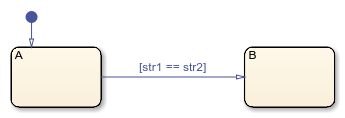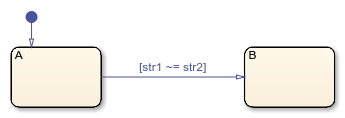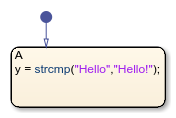# strcmp

Compare strings (case sensitive)

Since R2018b

## Syntax

``tf = strcmp(str1,str2)``
``tf = strcmp(str1,str2,n)``

## Description

example

````tf = strcmp(str1,str2)` compares strings `str1` and `str2`. In charts that use MATLAB® as the action language, the operator returns `1` (`true`) if the strings are identical and `0` (`false`) otherwise.In charts that use C as the action language, the operator returns `0` if the strings are identical. Otherwise, it returns a nonzero integer that depends on the compiler that you use. This value can differ in simulation and generated code. ```

example

````tf = strcmp(str1,str2,n)` compares the first `n` characters in `str1` and `str2`. NoteThis syntax is supported only in Stateflow® charts that use C as the action language. In charts that use MATLAB as the action language, use `strncmp`. ```

## Examples

expand all

Return a value of `1` (`true`) because the strings are equal.

`x = strcmp("Hello","Hello");`Return a value of `0` (`false`) because the strings are not equal.

`y = strcmp("Hello","Hello!");`You can compare strings by using relational operators. Use `==` to determine if two strings are equal.

`[str1 == str2]`Use `~=` to determine if two strings are not equal.

`[str1 ~= str2]`Return a value of `0` because the strings are equal.

`x = strcmp("Hello","Hello");`Return a nonzero value because the strings are not equal.

`y = strcmp("Hello","Hello!");`You can compare strings by using relational operators. Use `==` to determine if two strings are equal.

`[str1 == str2]`Use `!=` or `~=` to determine if two strings are not equal.

`[str1 != str2]`Return a value of `0` because the strings start with the same five characters.

`z = strcmp("Hello","Hello!",5);`This syntax is supported only in Stateflow charts that use C as the action language. In charts that use MATLAB as the action language, use `strncmp`.

## Input Arguments

expand all

Input strings, specified as string scalars. In charts that use MATLAB as the action language, enclose literal strings with double quotes.

Example: `"Hello"`

Number of characters checked, starting at the beginning of each string, specified as a positive integer.

## Version History

Introduced in R2018b